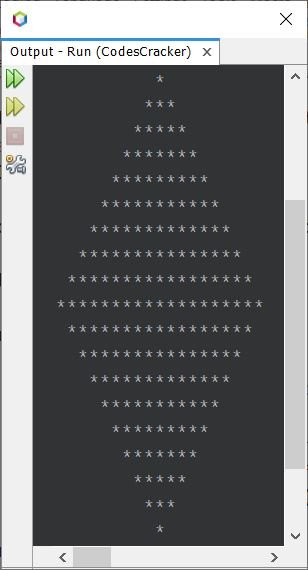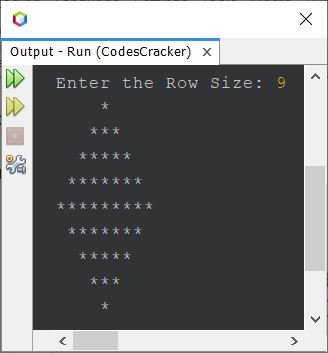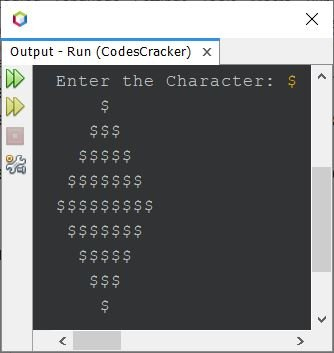# Java Program to Print Diamond Pattern

This article covers one of the important pattern program in Java, that is diamond pattern program. The diamond patterns are created here, using stars, numbers, alphabets, and any other desired character.

## Print Diamond Pattern of Stars in Java

The question is, write a Java program to print diamond pattern of stars. The program given below is its answer:

```public class CodesCracker
{
public static void main(String[] args)
{
int row=10, i, j, space;

for(i=0; i<row; i++)
{
for(space=i; space<(row-1); space++)
System.out.print(" ");
for(j=0; j<(i*2)+1; j++)
System.out.print("*");
System.out.print("\n");
}
for(i=0; i<(row-1); i++)
{
for(space=i; space>=0; space--)
System.out.print(" ");
for(j=(row*2)-3; j>(i*2); j--)
System.out.print("*");
System.out.print("\n");
}
}
}```

The snapshot given below shows the sample output produced by above Java program on printing of diamond using stars:Since the program given above prints the diamond pattern of stars, that doesn't match the row size. That is, I've given 10 as the row size, but the diamond pattern is printed with total of (row*2)-1 number of lines or rows. That is, 19 lines in this case.

Therefore, the program needs to be modified. So I've modified the program. Here is the new version of the above program, that does the same job as of previous, but cares about the value of variable row:

```public class CodesCracker
{
public static void main(String[] args)
{
int row=13, i, j, space, decider;
decider = row/2;

for(i=0; i<row; i++)
{
if(i<=decider)
{
for(space=i; space<decider; space++)
System.out.print(" ");
for(j=0; j<(i*2)+1; j++)
System.out.print("*");
System.out.print("\n");
}
else
{
for(space=i; space>decider; space--)
System.out.print(" ");
for(j=(row*2)-1; j>(i*2); j--)
System.out.print("*");
System.out.print("\n");
}
}
}
}```

The output produced by this program is:

```      *
***
*****
*******
*********
***********
*************
***********
*********
*******
*****
***
*```

### Print Diamond Pattern of Given Size using Stars in Java

This program allows user to define the size of diamond, at run-time of the program.

```import java.util.Scanner;

public class CodesCracker
{
public static void main(String[] args)
{
int row, i, j, space, decider;
Scanner s = new Scanner(System.in);

System.out.print("Enter the Row Size: ");
row = s.nextInt();

decider = row/2;

for(i=0; i<row; i++)
{
if(i<=decider)
{
for(space=i; space<decider; space++)
System.out.print(" ");
for(j=0; j<(i*2)+1; j++)
System.out.print("*");
System.out.print("\n");
}
else
{
for(space=i; space>decider; space--)
System.out.print(" ");
for(j=(row*2)-1; j>(i*2); j--)
System.out.print("*");
System.out.print("\n");
}
}
}
}```

Here is its sample run with user input 9 as row size, or number of lines, to expand the diamond of stars:Note - To print diamond pattern, provide only an odd value to the row.

## Print Diamond Pattern of Numbers in Java

This program is almost similar to second program. The only difference is, I've used num variable with its value as 1. You can try with any other number too. In this program, first the num variable is declared and initialized. And in the place of *, I've placed the num variable. That's it. Rest of the codes are similar to second program.

```public class CodesCracker
{
public static void main(String[] args)
{
int row=9, i, j, k, d, num=1;
d = row/2;

for(i=0; i<row; i++)
{
if(i<=d)
{
for(k=i; k<d; k++)
System.out.print(" ");
for(j=0; j<(i*2)+1; j++)
System.out.print(num);
System.out.print("\n");
}
else
{
for(k=i; k>d; k--)
System.out.print(" ");
for(j=(row*2)-1; j>(i*2); j--)
System.out.print(num);
System.out.print("\n");
}
}
}
}```

```    1
111
11111
1111111
111111111
1111111
11111
111
1```

## Print Diamond Pattern of Alphabets in Java

This program is almost similar to previous, as in place of num of int type, to print diamond of numbers, I've used alphabet of char type, to print diamond of alphabet.

```public class CodesCracker
{
public static void main(String[] args)
{
int row=9, i, j, k, d;
d = row/2;
char alphabet='A';

for(i=0; i<row; i++)
{
if(i<=d)
{
for(k=i; k<d; k++)
System.out.print(" ");
for(j=0; j<(i*2)+1; j++)
System.out.print(alphabet);
System.out.print("\n");
}
else
{
for(k=i; k>d; k--)
System.out.print(" ");
for(j=(row*2)-1; j>(i*2); j--)
System.out.print(alphabet);
System.out.print("\n");
}
}
}
}```

```    A
AAA
AAAAA
AAAAAAA
AAAAAAAAA
AAAAAAA
AAAAA
AAA
A```

## Print Diamond Pattern of any Given Character in Java

This is the last program of this article, created to allow user to enter the character to form the diamond using that character. The character can be anything such as 1, 2, R, O, s, b, #, %, }, [ etc.

```import java.util.Scanner;

public class CodesCracker
{
public static void main(String[] args)
{
int row=9, i, j, k, d;
d = row/2;
char ch;
Scanner s = new Scanner(System.in);

System.out.print("Enter the Character: ");
ch = s.next().charAt(0);

for(i=0; i<row; i++)
{
if(i<=d)
{
for(k=i; k<d; k++)
System.out.print(" ");
for(j=0; j<(i*2)+1; j++)
System.out.print(ch);
System.out.print("\n");
}
else
{
for(k=i; k>d; k--)
System.out.print(" ");
for(j=(row*2)-1; j>(i*2); j--)
System.out.print(ch);
System.out.print("\n");
}
}
}
}```

Here is its sample run with user input \$, a dollar sign or character#### Same Program in Other Languages

Java Online Test

« Previous Program Next Program »